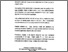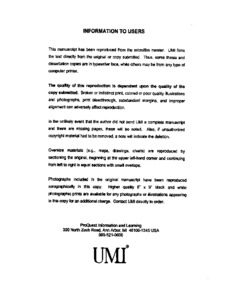Title:

Efficient enumeration of extensions of local fields with bounded discriminant

Pauli, Sebastian (2001) Efficient enumeration of extensions of local fields with bounded discriminant. PhD thesis, Concordia University.Preview
Text (application/pdf)
NQ63983.pdf
3MB

Abstract

Let k be a p -adic field. It is well-known that k has only finitely many extensions of a given finite degree. Krasner  gives formulae for the number of extensions of a given degree and discriminant. Following his work, we present an algorithm for the computation of generating polynomials for all extensions K / k of a given degree and discriminant. We also present canonical sets of generating polynomials of extensions of degree p m . Some methods from the proof of the number of extensions of a given degree and discriminant can also be used for the determination of a bound that gives a considerably improved estimate of the complexity of polynomial factorization over local fields. We use this bound in an efficient new algorithm for factoring a polynomial Z over a local field k . For every irreducible factor [varphi]( x ) of Z ( x ) our algorithm return an integral basis for k [ x ]/[varphi]( x ) k [ x ] over k .

Divisions: Concordia University > Faculty of Arts and Science > Mathematics and Statistics Thesis (PhD) Pauli, Sebastian viii, 96 leaves ; 29 cm. Concordia University Ph. D. Mathematics 2001 Ford, David 1533 Concordia University Library 27 Aug 2009 17:20 18 Jan 2018 17:17 http://clues.concordia.ca/search/c?SEARC...
All items in Spectrum are protected by copyright, with all rights reserved. The use of items is governed by Spectrum's terms of access.

Repository Staff Only: item control pageResearch related to the current document (at the CORE website)
Back to top